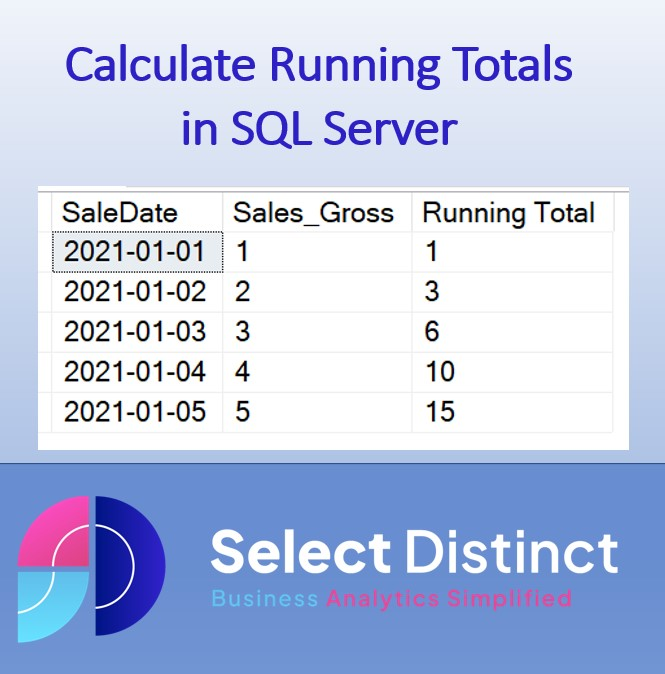## Blog# Running Totals in SQL Server

This blog post explains how to calculate running totals in SQL Server, why you would use them and provides a breakdown of the syntax

### What is a running total?

A running total is a cumulative sum that takes all the values of a sequence and adds them together. It is typically used in data analysis to calculate cumulative results over a period of time. For example, it can be used to calculate the running sum of sales over the past year. It can also be used to calculate a predicted closing inventory as part of an inventory forecasting process, taking into account predicted sales and expected deliveries.

### Why would you want to calculate a running total?

Calculating a running total can be helpful in many situations. It is an essential part of statistical and financial analysis and helps to explain trends, performance, and other changes over time. It is also helpful in forecasting sales and predicting future performance. Calculating running totals allows you to analyse data more effectively and find insights that would normally be hidden beneath the surface.
Using the inventory level prediction as an example, we could identify where there will be likely stock shortages. We can then use this information to bring in extra deliveries to keep up with demand at the right time

### How to calculate a running total in SQL Server

Let’s use a simple example to illustrate the steps
Assuming you have a table of daily sales records
We want to see the cumulative total of Sales at the end of each day
Using a ‘window function’ we can calculate the running total from the Sales (Gross) column
If we break down the elements of this code it will help to explain what each part does
You can now create running totals in SQL
From this code you can easily amend it to create running counts, running averages or other calculations

### Running Totals in SQL Server – Conclusion

In conclusion, running totals are an invaluable tool for data analysis, financial accounting, and forecasting. They are used to highlight cumulative values and give the perspective of what the balance was at that point in time
It is easy to calculate a running total in SQL Server using the SUM() function with the and the OVER clause. You can also bring in PARTITION to provide the ability to have separate running totals say for different departments, but we will show that in more detail in a future post
Watch our You Tube Video

Subscribe to our channel to see more Excel Timesavers